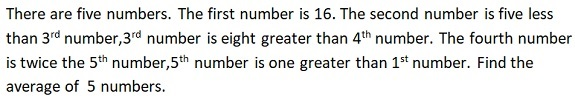Latest Results:

# 11plus Mean sample papers

Solve this Question

#### The mean of  5 numbers is 6. If we remove one of the numbers, the mean of the remaining numbers is 5. What is the number that was removed?

Solve this Question

#### Of three numbers, the first is double the second and the second is thrice the third. If their average is 40. Find the third number.

 1. 10 2. 14 3. 16 4. 12

Solve this Question

#### Find the average of all prime numbers between 30 and 50.

 1. 38.9 2. 36.8 3. 39.6 4. 39.8

Solve this Question

#### Find the mean of set of values 7, 7, 7 and 7.

 1. 4 2. 5.25 3. 6.5 4. 7

####